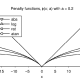# Star Puzzle

Here is an interesting challenge for you. The below mentioned puzzle may appear simple at first, but the solution may be little complex.

Programmers can do some coding to get the answer. If you do that, post it as a comment below. You can use ‘trial and error’ or any other method to get the answer. So here you go…

Sum on Each Line should be 26

A six-pointed star is drawn with six lines and twelve vertices. Arrange the integers 1 through 12, one on each vertex, so that the four integers on each line add to 26.

An example is given below but the numbers are wrong. The left-hand vertical line adds up correctly: 1+2+12+11=26, but the line 9+2+10+7=28 does not.I will post the answer on Saturday, 27 August, 2011.Till then keep trying. All the best 🙂#### Vineet Patawari

Hi, I'm Vineet Patawari. I fell in love with numbers after being scared of them for quite some time. Now, I'm here to make you feel comfortable with numbers and help you get rid of Math Phobia!

## 9 thoughts to “Star Puzzle”

1.aira says:

C’mon guys, tell me more! I almost got it. I like puzzles but I suck on Math puzzles.

1.mayya knn says:

My reply to the above STAR PUZZLE is as shown bellow a 10 b 4 7 9 6 c 8 5 d 1 11 12 2 e 3

2.Shanoor says:

Hey guyz! Plz let me know is dere any trick to solve dis puzzle, or it has to be solved by guess only???

1.mayya knn says:

Now we have to see how the numbers are to be placed. Let us assume the following; The sum of the numbers at the points is 26, while the total of all the numbers of the star is 78. Therefore, the sum of the numbers of the inner hexogen is 78-26=52 We shall now proceed onto examine one of the big tringles. The sum of the numbers on each of its sides is26. If we add up three sides we get 26 into3 is 78. But in this case , the numbers at the points will each be counted twice. Since the sum of the numbers of the three inner pairs-ie the inner hexogen- must,naturally be 52. Then the doubled sum at points of each triangle is 78-52=26 or 13 for each triangle. At this point our search narrows down. Weknow that neither 12 nor 11 can occupy the circles at the points. So wecan try 10 and immedeatly we come to the conclusion that the other two digits must be i and 2. It is all very simple now. All we have to do is follow up and eventually we shall find the exatct arrangement we are looking fur,as shown below Replacing the numbers of left hand vertical (from bottom to top ) 11,12,2,1. as 2,12,11,1., right hand vertical(from bottom to top ) 4,5,6,7. as 6,9,7,4 top diagonal line (from left to right) 9,2,10,7 as 1,8.7.10. bottom diagonal line (from left to right ) 11,3,5,8. as 3,12,5,6.

1.mayya knn says:

In the six pointed star in question , the replacement of the integers from 1 through 12 one on each vertex are shown within brackets ( ) as against in front of each integer, so that the four integers in each line add yo 26 as noted below 1 (4 ) , 2 ( 8 ) , 3 (12), 4(2), 5(5) , 6(9), 7(10), 8(6), 9(1), 10(7), 11(3), 12(11),.

3.Saptarshi pal chaudhuri says:

clockwise –>outer vertices 1 5 12 6 7 9 clockwise–>inner vertices 2 11 4 3 8 10 such that 2 lies next to 1 in clockwise fashion too….:)….
1+2+11+12=5+11+4+6=12+4+3+7=6+3+8+9=7+8+10+1=9+10+2+5=26

4.Tina says:

on outer six points- 8,9,7,5,11,12 (clockwise) and on inner points – 4,1,10,2,6,3 (clockwise) such that left hand line vertical is 8+4+3+11 and right hand vertical is 9+10+5+2

5.Mukesh Duggal says:

Outer six points- 1,2,7,3,4,5 (clockwise) and Inner points – 11,8,10,9,6,12 (clockwise) such that left hand vertical line (top to bottom) is 1,11,12,4 and right hand vertical line is 2,10,9,3 (top to bottom)

6.Chetna says:

on outer six points- 1,4,3,2,9,7 (clockwise) and on inner points – 10,12,8,6,11,5 (clockwise) such that left hand line vertical is 1+5+11+6 and right hand vertical is 1+10+12+3